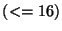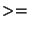IdentifiantMot de passe
Mot de passe oublié ?

# 7.3 Writing your own ufuncs!

This section describes a new process for defining your own universal functions. It explains a new interface that enables the description of N-ary ufuncs, those that use semi-arbitrary numbersof inputs and outputs.

## 7.3.1 Runtime components of a ufunc

A numarray universal function maps from a Python function name to a set of C functions. Ufuncs are polymorphic and figure out what to do in C when passed a particular set of input parameter types. C functions, on the other hand, can only be run on parameters which match their type signatures. The task of defining a universal function is one of describing how different parameter sequences are mapped from Python array types to C function signatures and back.

At runtime, there are three principle kinds of things used to define a universal function.

1. Ufunc

The universal function is itself a callable Python object. Ufuncs organize a collection of Cfuncs to be called based on the actual parameter types seen at runtime. The same Ufunc is typically associated with several Cfuncs each of which handles a unique Ufunc type signature. Because a Ufunc typically has more than one C func, it can also be implemented using more than one library function.

2. Library function

A pre-existing function written in C or Fortran which will ultimately be called for each element of the ufunc parameter arrays.

3. Cfunc

Cfuncs are binding objects that map C library functions safely into Python. It's the job of a Cfunc to interpret typeless pointers corresponding to the parameter arrays as particular C data types being passed down from the ufunc. Further, the Cfunc casts array elements from the input type to the Libraray function parameter type. This process lets the ufunc implementer describe the ufunc type signatures which will be processed most efficiently by the underlying Library function by enabling per-call element-by-element type casts. Ufunc calling signatures which are not represented directly by a Cfunc result in blockwise coercion to the closest matching Cfunc, which is slower.

## 7.3.2 Source components of a ufunc

There are 4 source components required to define numarray ufuncs, one of which is hand written, two are generated, and the last is assumed to be pre-existing:

1. Code generation script

The primary source component for defining new universal functions is a Python script used to generate the other components. For a standalone set of functions, putting the code generation directives in setup.py can be done as in the example numarray/Examples/ufunc/setup_airy.py. The code generation script only executes at install time.

2. Extension module

A private extension module is generated which contains a collection of Cfuncs for the package being created. The extension module contains a dictionary mapping from ufuncs/types to Cfuncs.

3. Ufunc init file

A Python script used at ufunc import time is required to construct Ufunc objects from Cfuncs. This code is boilerplate created with the code generation directive make_stub().

4. Library functions

The C functions which are ultimately called by a Ufunc need to be defined somewhere, typically in a third party C or Fortran library which is linked to the Extension module.

## 7.3.3 Ufunc code generation

There are several code generation directives provided by package numarray.codegenerator which are called at installation time to generate the Cfunc extension module and Ufunc init file.

 UfuncModule( module_name)
The UfuncModule constructor creates a module object which collects code which is later output to form the Cfunc extension module. The name passed to the constructor defines the name of the Python extension module, not the source code.
```m = UfuncModule("_na_special")
```

The add_code() method of a UfuncModule object is used to add arbitrary code to the module at the point that add_code() is called. Here it includes a header file used to define prototypes for the C library functions which this extension will ultimately call.
```m.add_code('#include "airy.h"')
```

 add_nary_ufunc( ufunc_name, c_name, ufunc_signatures, c_signature, forms=None)
The add_nary_ufunc() method declares a Ufunc and relates it to one library function and a collection of Cfunc bindings for it. The signatures parameter defines which ufunc type signatures receive Cfunc bindings. Input types which don't match those signature are blockwise coerced to the best matching signature. add_nary_ufunc() can be called for the same Ufunc name more than once and can thus be used to associate multiple library functions with the same Ufunc.
```m.add_nary_ufunc(ufunc_name = "airy",
c_function  = "airy",
signatures  =["dxdddd",
"fxffff"],
c_signature = "dxdddd")
```

 generate( source_filename)
The generate() method asks the UfuncModule object to emit the code for an extension module to the specified source_filename.
```m.generate("Src/_na_specialmodule.c")
```

The make_stub() function is used to generate the boilerplate Python code which constructs universal functions from a Cfunc extension module at import time. make_stub() accepts a add_code parameter which should be a string containing any additional Python code to be injected into the stub module. Here make_stub() creates the init file ``Lib/__init__.py'' associated with the Cfunc extension ``_na_special'' and includes some extra Python code to define the plot_airy() function.
```extra_stub_code = '''

import matplotlib.pylab as mpl

def plot_airy(start=-10,stop=10,step=0.1,which=1):
a = mpl.arange(start, stop, step)
mpl.plot(a, airy(a)[which])

b = 1.j*a + a
ba = airy(b)[which]

h = mpl.figure(2)
mpl.plot(b.real, ba.real)

i = mpl.figure(3)
mpl.plot(b.imag, ba.imag)

mpl.show()
'''

```

## 7.3.4 Type signatures and signature ordering

Type signatures are described using the single character typecodes from Numeric. Since the type signature and form of a Cfunc need to be encoded in its name for later identification, it must be brief.

```typesignature ::= <inputtypes> + ``x'' + <outputtypes>
inputtypes ::= [<typecode>]+
outputtypes ::= [<typecode>]+
typecode ::= "B" | "1" | "b" | "s" | "w" | "i" | "u" |
"N" | "U" | "f" | "d" | "F" | "D"
```

For example, the type signature corresponding to one Int32 input and one Int16 output is "ixs".

A type signature is a sequence of ordered types. One signature can be compared to another by comparing corresponding elements, in left to right order. Individual elements are ranked using the order from the previous section. A ufunc maintains its associated Cfuncs as a sorted sequence and selects the first Cfunc which isthe input type signature; this defines the notion of ``best matching''.

## 7.3.5 Forms

The add_nary_ufunc() method has a parameter forms which enables generation of code with some extra properties. It specifies the list of function forms for which dedicated code will be generated. If you don't specify forms, it defaults to a (list of a) single form which specifies that all inputs and outputs corresponding to the type signature are vectors. Input vectors are passed by value, output vectors are passed by reference. The default form implies that the library function return value, if there is one, is ignored. The following Python code shows the default form:

```["v"*n_inputs + "x" + "v"*n_outputs]
```

Forms are denoted using a syntax very similar to, and typically symmetric with, type signatures.

```form ::=  <inputs> "x" <outputs>
inputs ::= ["v"|"s"]*
outputs ::= ["f"]?["v"]* | "A" | "R"

The form character values have different meanings than for type
signatures:

'v'  :   vector,  an array of input or output values
's'  :   scalar,  a non-array input value
'f'  :   function,  the c_function returns a value
'R'  :   reduce,    this binary ufunc needs a reduction method
'A'  :   accumulate this binary ufunc needs an accumulate method
'x'  :   separator  delineates inputs from outputs
```

So, a form consists of some input codes followed by a lower case "x" followed by some output codes.

The form for a C function which takes 4 input values, the last of which is assumed to be a scalar, returns one value, and fills in 2 additional output values is: "vvvsxfvv".

Using "s" to designate scalar parameters is a useful performance optimization for cases where it is known that only a single value is passed in from Python to be used in all calls to the c function. This prevents the blockwise expansion of the scalar value into a vector.

Use "f" to specify that the C function return value should be kept; it must always be the first output and will therefore appear as the first element of the result tuple.

For ufuncs of two input parameters (binary ufuncs), two additional form characters are possible: A (accumulate) and R (reduce). Each of these characters constitutes the *entire* ufunc form, so the form is denoted "R" or "A". For these kinds of cfuncs, the type signature always reads `<t>x<t>` where `<t>` is one of the type characters.

One reason for all these codes is so that the many Cfuncs generated for Ufuncs can be easily named. The name for the Cfunc which implements add() for two Int32 inputs and one Int32 output and where all parameters are arrays is: "add_iixi_vvxv". The cfunc name for add.reduce() with two integer parameters would be written as "add_ixi_R" and for add.accumulate() as "add_ixi_A".

The set of Cfuncs generated is based on the signatures crossed with the forms. Multiple calls to add_nary_ufunc() can be used the reduce the effects of signature/form crossing.

## 7.3.6 Ufunc Generation Example

This section includes code from Examples/ufunc/setup_airy.py in the numarray source distribution to illustrate how to create a package which defines your own universal functions.

This script eventually generates two files: _na_airymodule.c and __init__.py. The former defines an extension module which creates numarray cfuncs, c helpers for the numarray airy() ufunc. The latter file includes Python code which automatically constructs numarray universal functions (ufuncs) from the cfuncs in _na_airymodule.c.

```import distutils, os, sys
from distutils.core import setup
from numarray.codegenerator import UfuncModule, make_stub
from numarray.numarrayext import NumarrayExtension

m = UfuncModule("_na_special")

c_function  = "airy",
signatures  =["dxdddd",
"fxffff"],
c_signature = "dxdddd")

c_function  ="cairy_fake",
signatures  =["DxDDDD",
"FxFFFF"],
c_signature = "DxDDDD")

m.generate("Src/_na_specialmodule.c")
```

```extra_stub_code = '''
def plot_airy(start=-10,stop=10,step=0.1,which=1):
import matplotlib.pylab as mpl;

a = mpl.arange(start, stop, step);
mpl.plot(a, airy(a)[which]);

b = 1.j*a + a
ba = airy(b)[which]

h = mpl.figure(2)
mpl.plot(b.real, ba.real)

i = mpl.figure(3)
mpl.plot(b.imag, ba.imag)

mpl.show()
'''

make_stub("Lib/__init__", "_na_special",

dist = setup(name = "na_special",
version = "0.1",
maintainer = "Todd Miller",
maintainer_email = "jmiller@stsci.edu",
description = "airy() universal function for numarray",
url = "http://www.scipy.org/",
packages = ["numarray.special"],
package_dir = { "numarray.special":"Lib" },
ext_modules = [ NumarrayExtension( 'numarray.special._na_special',
['Src/_na_specialmodule.c',
'Src/airy.c',
'Src/const.c',
'Src/polevl.c']
)
]
)
```

Additional explanatory text is available in numarray/Examples/ufunc/setup_airy.py. Scripts used to extract numarray ufunc specs from the existing Numeric ufunc definitions in scipy.special are in numarray/Examples/ufunc/RipNumeric as an example of how to convert existing Numeric code to numarray.

Send comments to the NumArray community.